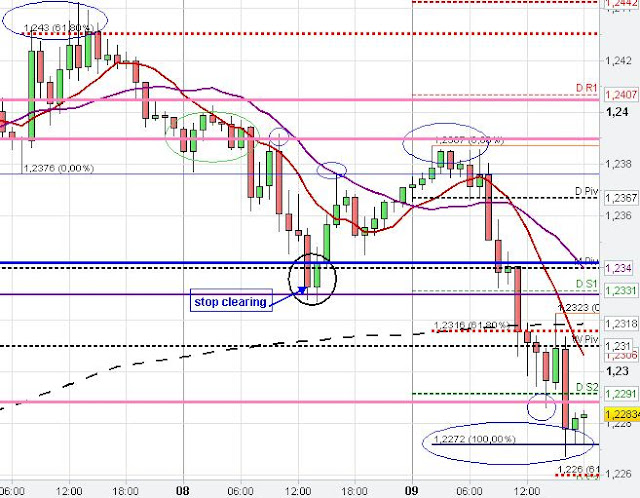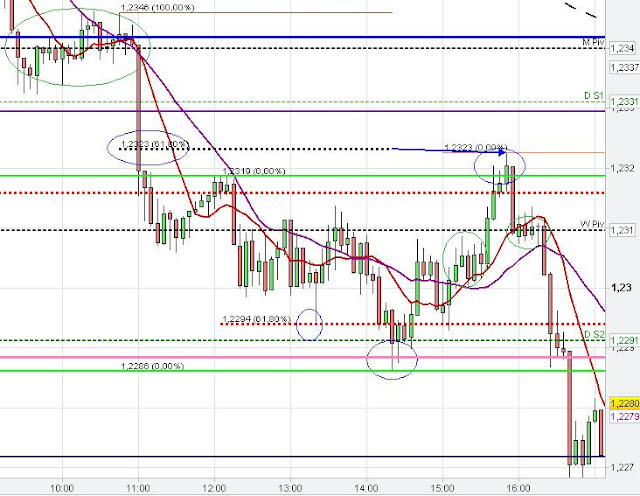## Risk Disclaimer

Risk Disclaimer: Trading is risky! Never trade money you can not afford to lose! Site content is only personal opinion and never a Trade Recommendation!

# Fibonacci Analysis

## Fibonacci Retracements and Fibonacci Extensions

### Understanding Fibonacci Trading

Fibonacci Retracement means the percentage depth of the retracement in relation to the prior swing. The major level used here is the 61.80 % Fibonacci Retracement level of the recent swing. A minor Fibonacci level is the 38.2 % Fibonacci Retracment level (not used here)

Fibonacci Extension is the length of the last swing moved to/ projected from the succeeding retracement/ correction level in direction of the last swing, with either the 100 % length or the 61.80 % length of the last swing. Thus, the projection from a major swing in direction of this swing is taken from the largest retracement level, reached by the succeeding correcting of this major swing to reveal hidden support and resistance levels.

There is also another type of Fibonacci Extension, which gives the 127 % and 161 % Extension of a swing without moving it to the succeeding retracement level. However, this kind of Fibonacci Extension is not meant or used here.

Fibonacci study: swing study and trend analysis chapter is below5 min 61.80 % Fibonacci Retracement1 hour Fibonacci Extension5 min Fibonacci Retracement and Extension

### Fibonacci Extension Levels

#### Swing Study and Trend Analysis

In general, if a trend prevails then market often moves strongly in one direction then consolidates before market resumes the trend. Typical price targets of the trend continuation are the 61.80 % (weak), 100 % (normal) and 161 % (strong) Fibonacci Extensions. The initial target is often the 61.80 % Fibonacci Extension target where market price often temporarily reacts. However, in a strong trend market could easily ignore the 61.80 % Fibonacci Extension level or only react on a shorter time frame and proceed directly to the major 100 Fibonacci Extension level which might provide some temporary or permanent Support/ Resistance.

Very often price consolidates (with smaller consolidation pattern than the prior consolidation) (bear/ bull flag) at the 61.80 % Fibonacci Extension before the trend continues to the 100 % Fibonacci Extension level. However, when price is not at least consolidating at the 61.80 % Fibonacci Extension level and instead bouncing back strongly into the prior consolidation (V-shape) then the underlying trend might be in question and a market price turn around or a larger consolidation pattern is likely to occur.

Furthermore, the last price breakout is often a false one to catch stop loss orders and to fool breakout traders, particularly at strong Support/ Resistance levels, which hold or only temporarily getting breached without any price confirmation.Fibonacci Extension levels and Trend pattern

Market report to the chart above:

On the chart above we have a downward trend. We see how the use of the 61.80 % and 100 % Fibonacci Extension levels provided some interesting price targets for the forex trader.

After the swing down (A-B) in the European Session the EUR/USD consolidated (typical 3 wave consolidation) and the Euro found resistance at the decreasing 20 SMA and the 1.28 round number. The Euro dropped down further (C-D) and the EUR/USD found some support at the Weekly S2 and the 61.80 % Fibonacci Extension from the start of the sudden drop in price from A to B at C at about 1.2757.

The 61.80 % Fibonacci Extension level is very often only intermediate support/ resistance particularly in a strong trend where price sometimes reacts/ consolidates before the market goes further to the major 100 % Fibonacci Extension level.

The EUR/USD price dropped down again and reached the 100 % Fibonacci Extension (A-B at C) and the 100 Fibonacci Extension from C-D at E at about 1.2729.

Below is another Chart example for the trend analysis using Fibonacci Extension levels but without chart explanation:Fibonacci Extensions/ Trend